# Direct and Inverse Proportion

## A self-marking exercise in three levels on solving direct and inverse variation problems.

##### Level 1Level 2Level 3Unitary MethodDescriptionHelpExam-StyleMore Ratio

This is level 1; Direct proportion. You can earn a trophy if you get at least 9 correct.

 1. If $$a$$ varies directly with $$b$$ and $$a=20$$ when $$b=4$$       find $$a$$ when $$b=7$$and find $$b$$ when $$a=45$$2. If $$c$$ varies directly with $$d$$ and $$c=21$$ when $$d=3$$       find $$c$$ when $$d=7$$and find $$d$$ when $$c=35$$3. If $$e$$ varies directly with $$f$$ and $$e=70$$ when $$f=7$$       find $$e$$ when $$f=8$$and find $$f$$ when $$e=120$$4. If $$g$$ varies directly with $$h$$ and $$g=14.8$$ when $$h=4$$       find $$g$$ when $$h=6$$and find $$h$$ when $$g=37$$5. The distance travelled by a train is directly proportional to the time taken.If the train can travel 435 kilometers in 5 hours find how many kilometers it can travel in 7 hours.6. The cost of a drain pipe is directly proportional to its length.A pipe costing £22.08 is 48 centimetres long. How many centimetres long would a drain pipe costing £33.58 be?7. If the pressure of the water on a diver at any point below the surface of the sea varies as the depth of the diver below the surface. If the pressure is 15 psi at a depth of 10 metres, calculate the pressure (in psi) at a depth of 18 metres.8. Click or tap on the graph which could represent direct proportion. A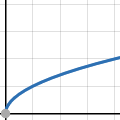B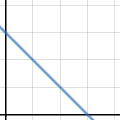C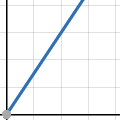D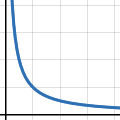E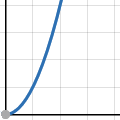Check

This is Direct and Inverse Proportion level 1. You can also try:
Level 2 Level 3

## Instructions

Try your best to answer the questions above. Type your answers into the boxes provided leaving no spaces. As you work through the exercise regularly click the "check" button. If you have any wrong answers, do your best to do corrections but if there is anything you don't understand, please ask your teacher for help.

When you have got all of the questions correct you may want to print out this page and paste it into your exercise book. If you keep your work in an ePortfolio you could take a screen shot of your answers and paste that into your Maths file.## More Activities:

Mathematicians are not the people who find Maths easy; they are the people who enjoy how mystifying, puzzling and hard it is. Are you a mathematician?

Comment recorded on the 2 May 'Starter of the Day' page by Angela Lowry, :

"I think these are great! So useful and handy, the children love them.
Could we have some on angles too please?"

Comment recorded on the 5 April 'Starter of the Day' page by Mr Stoner, St George's College of Technology:

"This resource has made a great deal of difference to the standard of starters for all of our lessons. Thank you for being so creative and imaginative."

Each month a newsletter is published containing details of the new additions to the Transum website and a new puzzle of the month.

The newsletter is then duplicated as a podcast which is available on the major delivery networks. You can listen to the podcast while you are commuting, exercising or relaxing.

Transum breaking news is available on Twitter @Transum and if that's not enough there is also a Transum Facebook page.

##### Featured ActivityThis spectacular magic trick never fails to amaze people of all ages assuming they can add and subtract very simple numbers. The mathematics comes in finding out how the trick works.

There are answers to this exercise but they are available in this space to teachers, tutors and parents who have logged in to their Transum subscription on this computer.

A Transum subscription unlocks the answers to the online exercises, quizzes and puzzles. It also provides the teacher with access to quality external links on each of the Transum Topic pages and the facility to add to the collection themselves.

Subscribers can manage class lists, lesson plans and assessment data in the Class Admin application and have access to reports of the Transum Trophies earned by class members.

Subscribe

## Go Maths

Learning and understanding Mathematics, at every level, requires learner engagement. Mathematics is not a spectator sport. Sometimes traditional teaching fails to actively involve students. One way to address the problem is through the use of interactive activities and this web site provides many of those. The Go Maths page is an alphabetical list of free activities designed for students in Secondary/High school.

## Maths Map

Are you looking for something specific? An exercise to supplement the topic you are studying at school at the moment perhaps. Navigate using our Maths Map to find exercises, puzzles and Maths lesson starters grouped by topic.

## Teachers

If you found this activity useful don't forget to record it in your scheme of work or learning management system. The short URL, ready to be copied and pasted, is as follows:

Alternatively, if you use Google Classroom, all you have to do is click on the green icon below in order to add this activity to one of your classes.

It may be worth remembering that if Transum.org should go offline for whatever reason, there is a mirror site at Transum.info that contains most of the resources that are available here on Transum.org.

When planning to use technology in your lesson always have a plan B!

Monday, September 2, 2019

Do you have any comments? It is always useful to receive feedback and helps make this free resource even more useful for those learning Mathematics anywhere in the world. Click here to enter your comments.For All:

## Description of Levels

CloseLevel 1 - Direct proportion

Level 2 - Inverse proportion

Level 3 - Mixed non-linear questions

Unitary Method - Test your understanding of the Unitary Method for solving real life proportion problems with this online, self-marking quiz.

Exam Style Questions - A collection of problems in the style of GCSE or IB/A-level exam paper questions (worked solutions are available for Transum subscribers).

More on this topic including lesson Starters, visual aids, investigations and self-marking exercises.

Answers to this exercise are available lower down this page when you are logged in to your Transum account. If you don’t yet have a Transum subscription one can be very quickly set up if you are a teacher, tutor or parent.

## Direct and Inverse Proportion

This video is from Mannel's Maths Music.

### Level 1 Example

If $$a$$ varies directly with $$b$$ and $$a=24$$ when $$b=8$$ find $$a$$ when $$b=9$$

$$a \propto b$$ $$a = kb$$

Where $$k$$ is some constant. If $$a=24$$ when $$b=8$$ then

$$24 = 8k$$ $$k = 3$$

so the equation is

$$a = 3b$$

If $$b = 9$$ then

$$a = 3 \times 9 = 27$$

### Level 2 Example

If $$a$$ is inversely proportional to $$b$$ and $$a=4$$ when $$b=6$$ find $$a$$ when $$b=8$$

$$a \propto \frac{1}{b}$$ $$a = \frac{k}{b}$$

Where $$k$$ is some constant. If $$a=4$$ when $$b=6$$ then

$$4 = \frac{k}{6}$$ $$k = 24$$

so the equation is

$$a = \frac{24}{b}$$

If $$b = 8$$ then

$$a = 24 \div 8 = 3$$

### Level 3 Example

If $$a$$ is directly proportional to the square of $$b$$ and $$a=24$$ when $$b=2$$ find $$a$$ when $$b=3$$

$$a \propto b^2$$ $$a = kb^2$$

Where $$k$$ is some constant. If $$a=24$$ when $$b=2$$ then

$$24 = 2^2 \times k$$ $$k = 6$$

so the equation is

$$a = 6b^2$$

If $$b = 3$$ then

$$a = 6 \times 3^2 = 54$$

Don't wait until you have finished the exercise before you click on the 'Check' button. Click it often as you work through the questions to see if you are answering them correctly. You can double-click the 'Check' button to make it float at the bottom of your screen.

Answers to this exercise are available lower down this page when you are logged in to your Transum account. If you don’t yet have a Transum subscription one can be very quickly set up if you are a teacher, tutor or parent.

Close